# Different Forms Of The Equation Of Line

We know that there are infinite points in the coordinate plane. Consider an arbitrary point P(x,y) on the XY plane and a line L. How will we confirm whether the point is lying on line L? This is where the importance of the equation of a straight line comes into the picture in two-dimensional geometry.

The equation of a straight line contains terms in x and y. If the point P(x,y) satisfies the equation of the line, then the point P lies on the line L.

## Different forms of equations of a straight line

1. Equations of horizontal and vertical lines

Equation of the lines which are horizontal or parallel to the X-axis is y = a, where a is the y – coordinate of the points on the line.

Similarly, the equation of a straight line which is vertical or parallel to the Y-axis is x = a, where a is the x-coordinate of the points on the line.

For example, the equation of the line which is parallel to the X-axis and contains the point (2,3) is y= 3.

Similarly, the equation of the line which is parallel to the Y-axis and contains the point (3,4) is x = 3.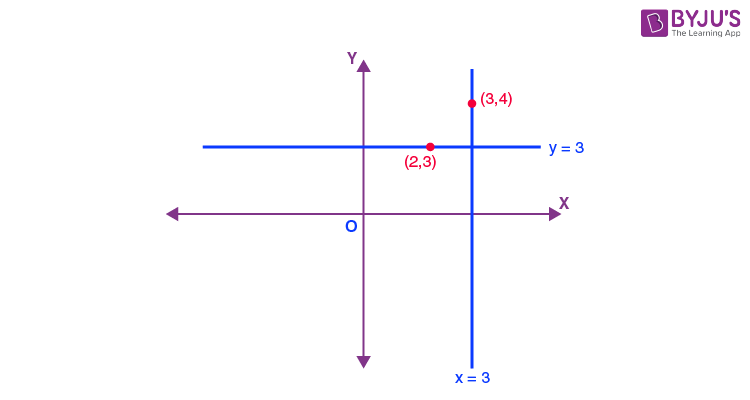2. Point-slope form equation of a line

Consider a non-vertical line L whose slope is m, A(x,y) be an arbitrary point on the line and  P(x1, y1) be the fixed point on the same line.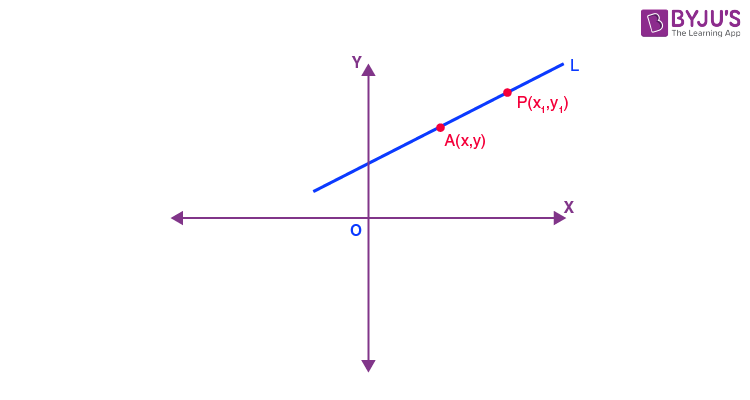Slope of the line by the definition is,

$$\begin{array}{l}m = \frac{y~-~y_1}{x~-~x_1}\end{array}$$
$$\begin{array}{l}y~-~y_1 = m(x~-~x_1)\end{array}$$

For example, equation of the straight line having a slope m = 2 and passes through the point (2, 3) is

y – 3 = 2(x – 2)

y= 2x-4+3

2x-y-1 = 0

3. Two-point form equation of line

Let P(x,y) be the general point on the line L which passes through the points A(x1, y1) and B(x2, y2).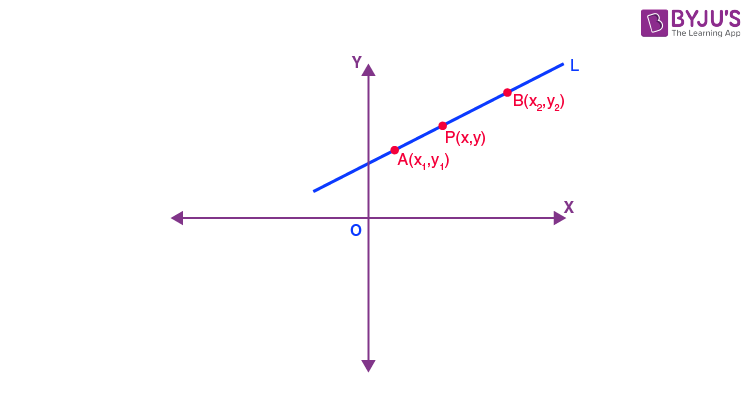Since the three points are collinear,

slope of PA = slope of AB

$$\begin{array}{l}\frac{y~-~y_1}{x~-~x_1} = \frac{y_2~-~y_1}{x_2~-~x_1}\end{array}$$
$$\begin{array}{l}y – y_{1} = (y_{2} – y_{1}). \frac{x – x_{1} }{x_{2} – x_{1}}\end{array}$$

4. Slope-intercept form equation of line

Consider a line whose slope is m which cuts the Y-axis at a distance ‘a’ from the origin. Then the distance a is called the y-intercept of the line. The point at which the line cuts the y-axis will be (0, a).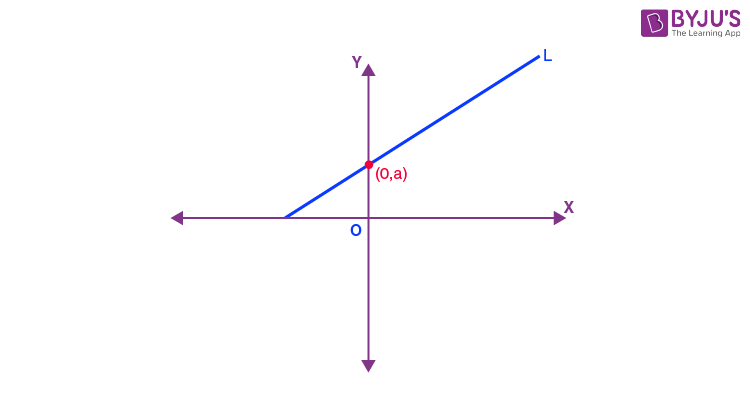Then, equation of the line will be

y-a = m(x-0)

y = mx+a

Similarly, a straight line having slope m cuts the X-axis at a distance b from the origin will be at the point (b,0). The distance b is called x- intercept of the line.

Equation of the line will be:

y = m(x-b)

5. Intercept form

Consider a line L having x– intercept a and y– intercept b, then the line touches X– axis at (a,0) and Y– axis at (0,b).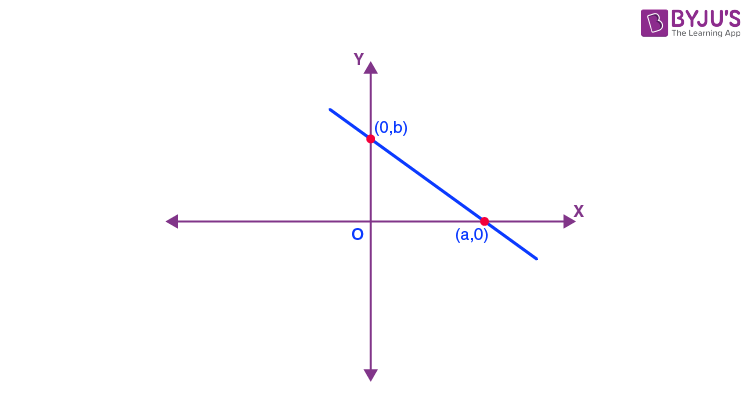By two-point form equation,

$$\begin{array}{l}y~-~0 = \frac{b-0}{0~-~a} (x~-~a)\end{array}$$
$$\begin{array}{l}y= -\frac{b}{a} (x~-~a)\end{array}$$
$$\begin{array}{l}y = \frac{b}{a} (a~-~x)\end{array}$$
$$\begin{array}{l}\frac{x}{a} ~+ ~\frac{y}{b} = 1\end{array}$$

For example, equation of the line which has x– intercept 3 and y– intercept 4 is,

$$\begin{array}{l}\frac{x}{3} ~+ ~\frac{y}{4} = 1\end{array}$$
$$\begin{array}{l}4x~ + ~3y = 12\end{array}$$

6. Normal form

Consider a perpendicular from the origin having length l to line L and it makes an angle β with the positive X-axis.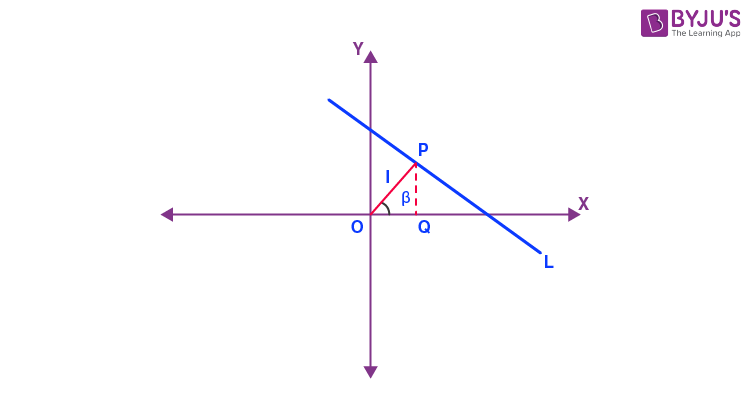Let OP be perpendicular from the origin to line L.
Then,

$$\begin{array}{l}OQ = l~ cos \beta \end{array}$$
$$\begin{array}{l}PQ = l~ sin\beta \end{array}$$

Coordinates of the point Pare;

$$\begin{array}{l}P(l ~cos~\beta ,l ~sin~\beta )\end{array}$$

The slope of the line OP is tan β

Therefore,

$$\begin{array}{l}Slope~ of ~the ~line ~L = -\frac{1}{tan~\beta}=-\frac{cos~\beta}{sin~\beta }\end{array}$$

Equation of the line L having slope -cos β / sin β and passing through the point (l cos β, l sin β) is,

$$\begin{array}{l}y~-~l~ sin~\beta = -\frac{cos~\beta }{sin~\beta } (x~-~l ~cos\beta )\end{array}$$
$$\begin{array}{l}y ~sin~\beta ~-~l~ sin^2~ \beta = -x ~cos~\beta ~+~l~cos^2~\beta \end{array}$$
$$\begin{array}{l}x~ cos~\beta ~ + ~y ~sin~\beta = l(sin^2~\beta ~+ ~cos^2~\beta )\end{array}$$
$$\begin{array}{l}x ~cos~\beta ~ + ~y ~sin~\beta = l\end{array}$$

You have learnt about the different forms of the equation of a straight line. To know more about straight lines and their properties, register with BYJU’S – The Learning App.